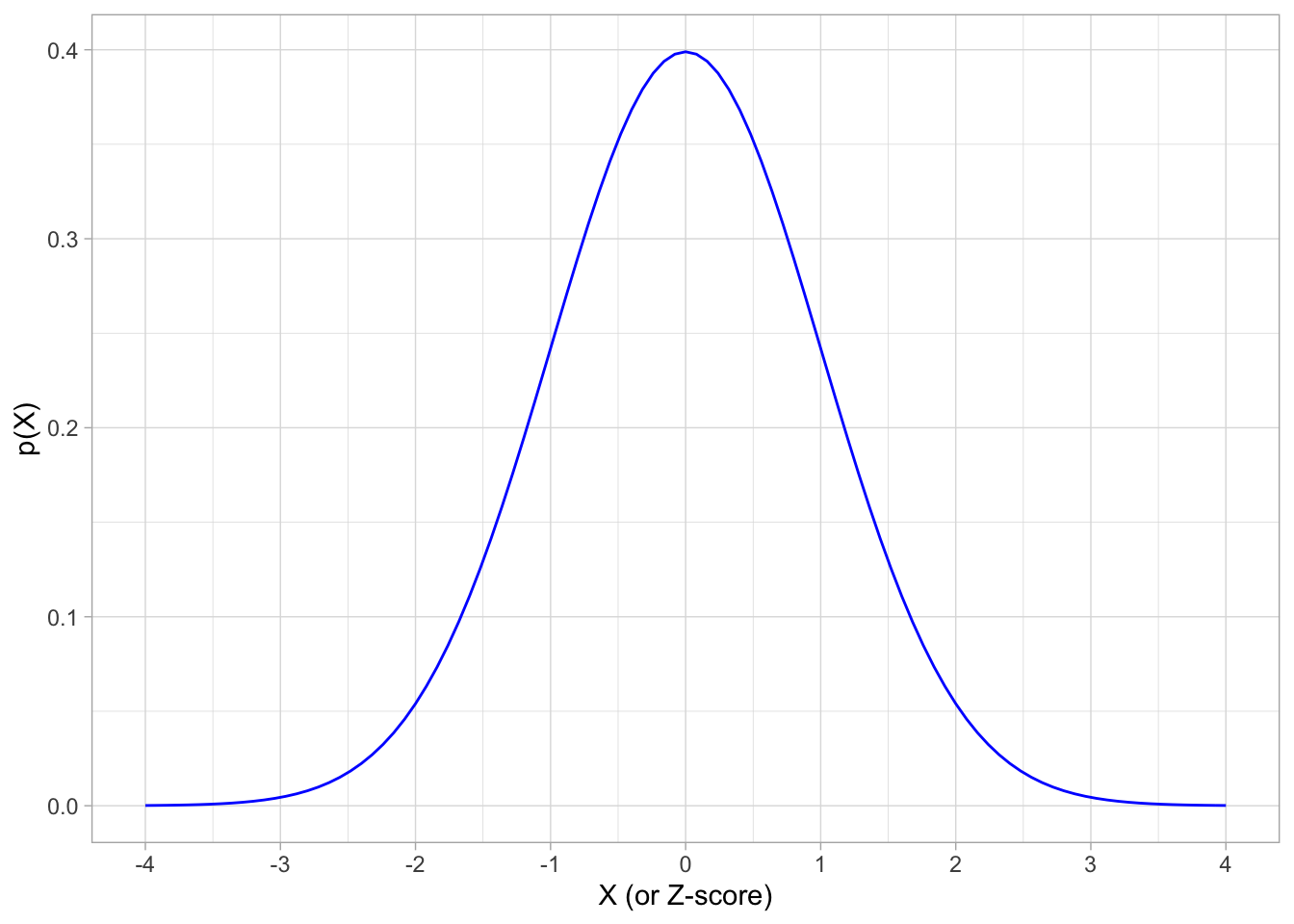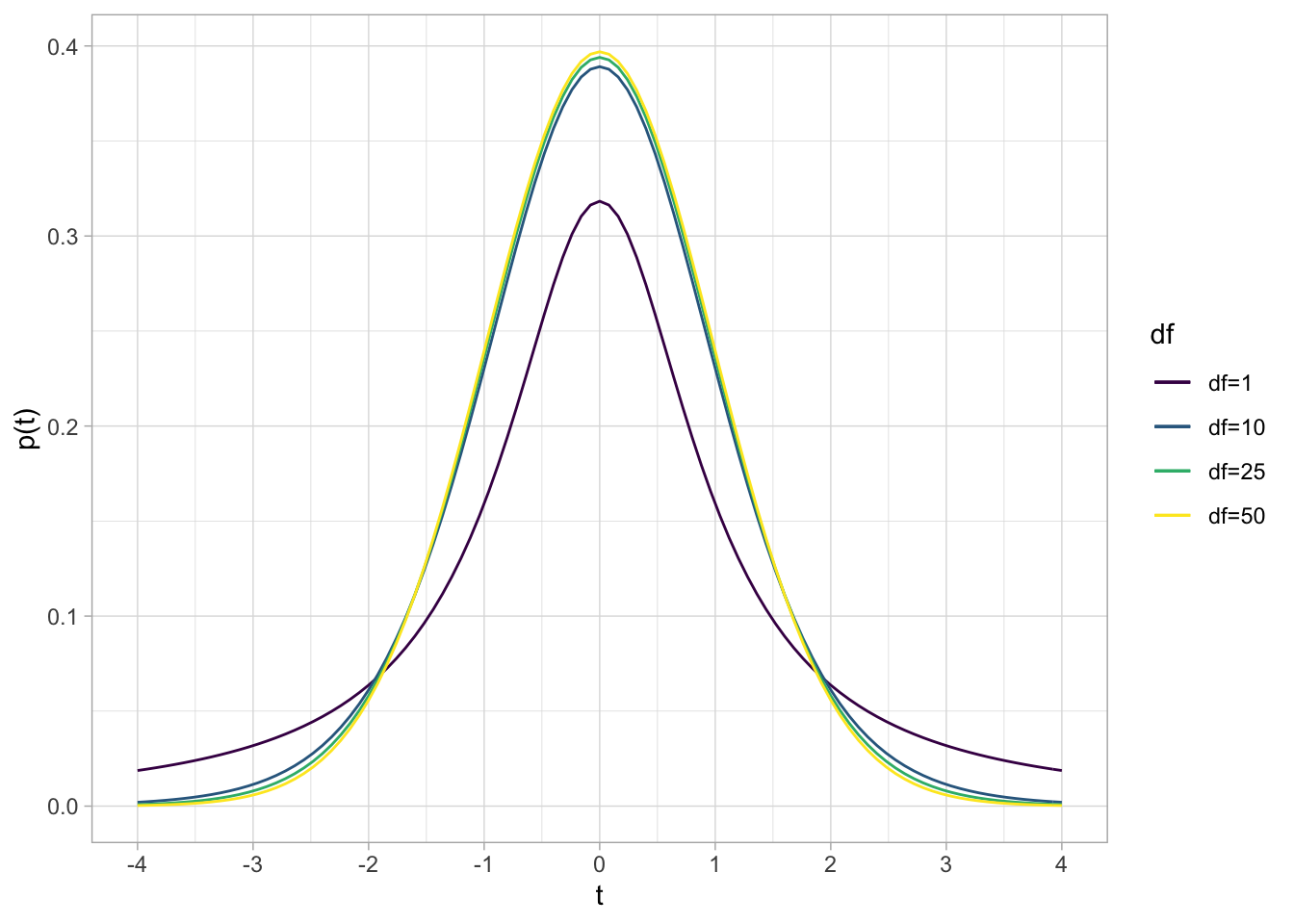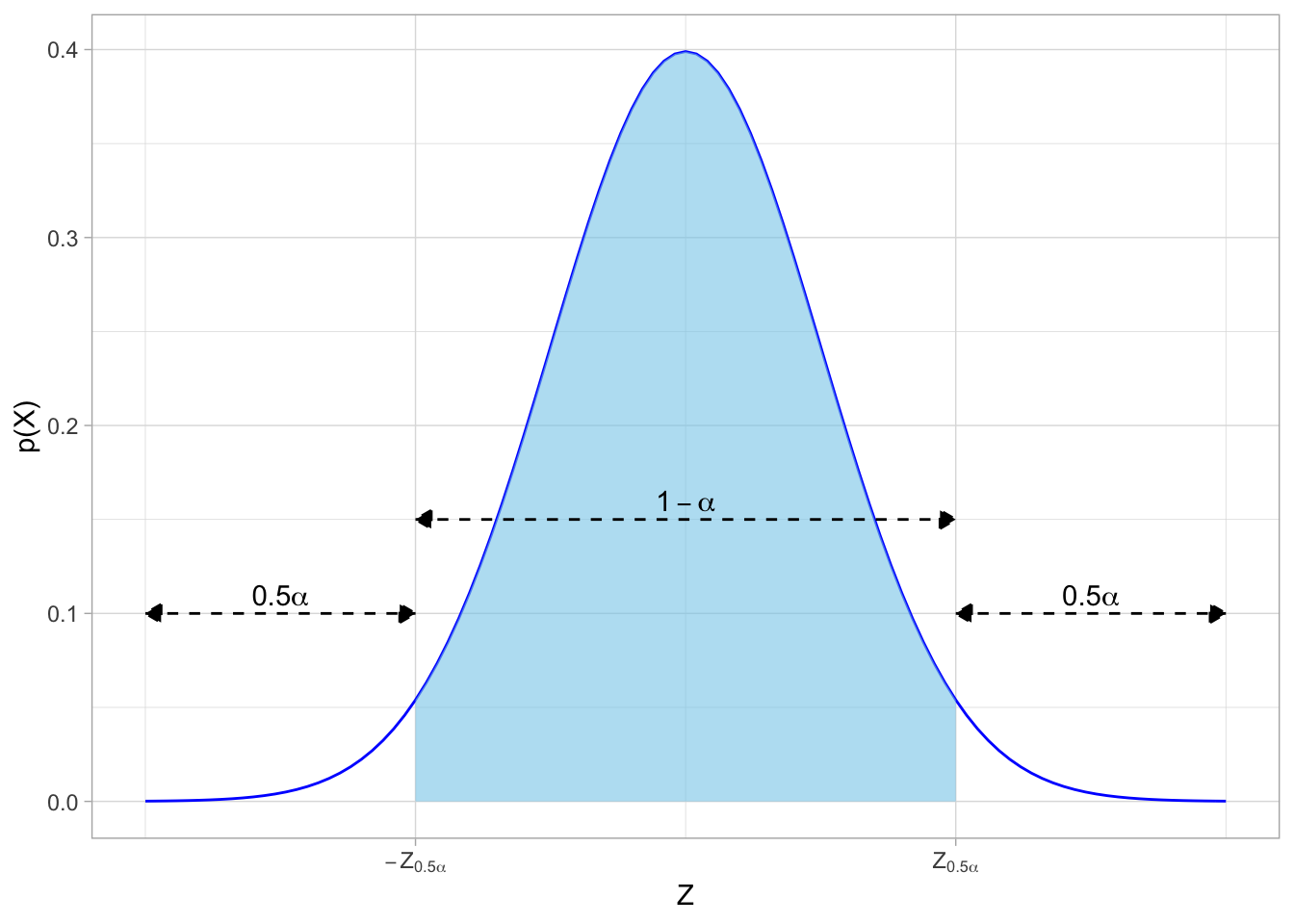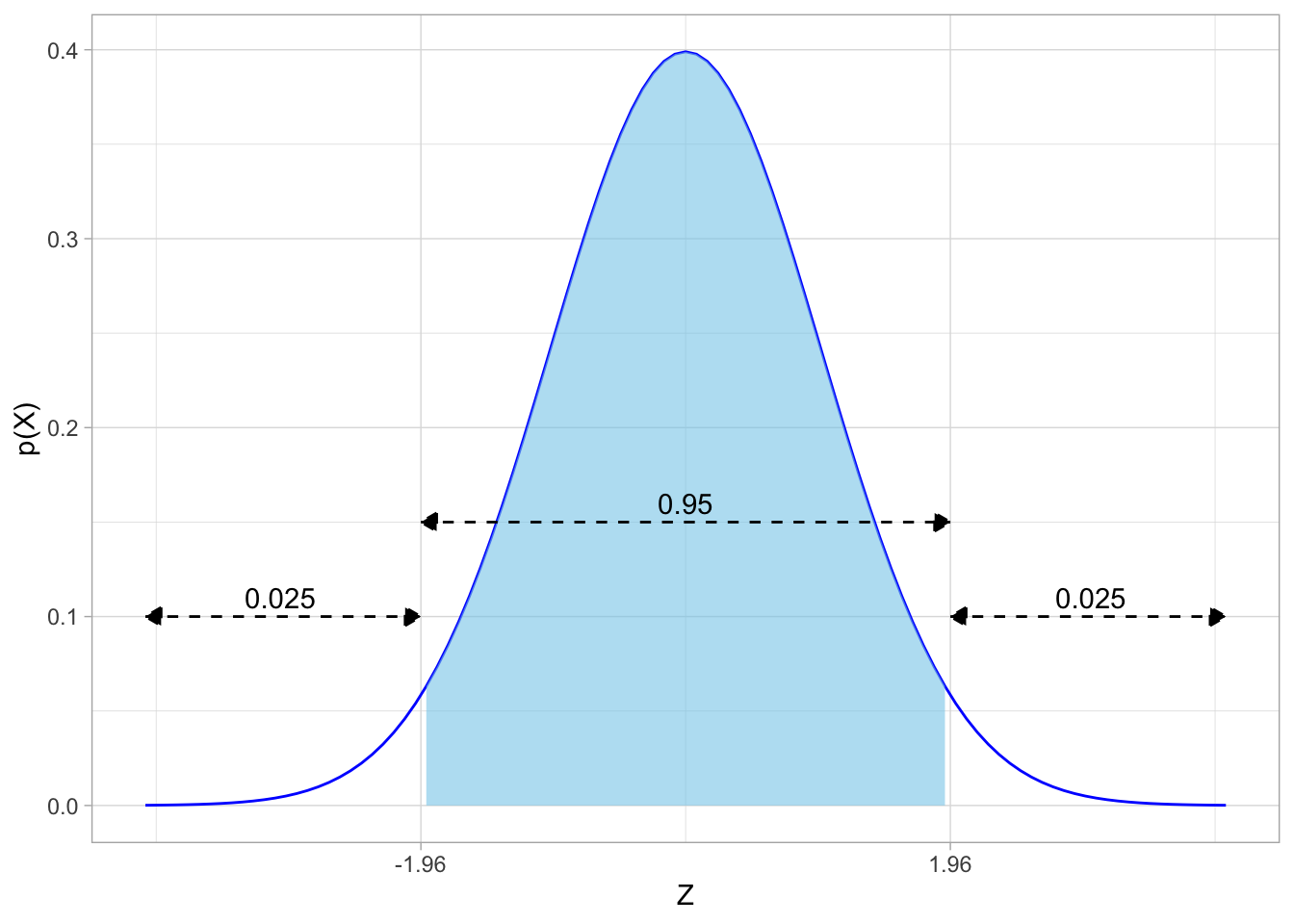# 2.6 — Statistical Inference — Class Notes

## Contents

Tuesday, September 22, 2020

## Overview

We begin with some more time for you to work on the R Practice from last class.

This class will be spread over 2 days, and is about inferential statistics: using statistics calculated from a sample of data to infer the true (and unmeasurable) parameters that describe a population. In doing so, we can run hypothesis tests on our sample to determine a point estimate of a parameter, or construct a confidence interval from our sample to cast a range for the true parameter.

This is standard principles of statistics - you hopefully should have learned it before. If it has been a while (or never) since your last statistics class, this is one of the hardest concepts to understand at first glance. I recommend Khan AcademyFrom sampling distributions through significance tests, for this. Though the whole class is helpful!

or Google for these concepts, as every statistics class will cover them in the standard way.

That being said, this semester I am not going to cover them in the standard way (see the appendix today below for an overview of the standard way). I think it will be more intuitive if I show you where these concepts come from by simulation as opposed to theoretical sampling distributions.

## Live Class Session on Zoom

The live class Zoom meeting link can be found on Blackboard (see LIVE ZOOM MEETINGS on the left navigation menu), starting at 11:30 AM.

If you are unable to join today’s live session, or if you want to review, you can find the recording stored on Blackboard via Panopto (see Class Recordings on the left navigation menu).

## Practice Problems

You will be finishing up R practice problems from last class. Answers are posted on that page.

## Assignments: Problem Set 3 & Midterm Exam

Problem Set 3 is posted and is due by 11:59 PM Sunday September 27 by upload on Blackboard. Please see that page for more information on how to submit (there are multiple ways!).

The midterm exam (on 1.1-2.7) will be next week. I will provide more information this week. We will dedicate time for review and questions on class Tuesday, September 29

## New Packages Mentioned

• infer: for simulation for statistical inference

## Assignments: Problem Set 3 Due Sunday September 27, Midterm

Problem Set 3 is due by 11:59PM on Sunday, September 27 by PDF upload to Blackboard.

Exam 1 (on 1.1-2.7) will be next week (week of September 28), I will provide more details next class. We save some time for review on Tuesday, September 29

## Inferential Statistics

### Differences Between What We Learned in Class and Classical Statistics

In class, you learned the basics behind inferential statistics–p-values, confidence intervals, hypothesis testing–via empirical simulation of many samples permuted from our existing data. We took our sample, ran 1,000 simulations by permutation of our sample without replacementThat is, for each simulation, we randomly selected observations from our existing sample to be in the simulation, and then did not put that observation back in the pool to possibly be selected again.

, calculated the statistic ($$\hat{\beta}_0$$, the slope) of each simulation; this gave us a (sampling) distribution of our sample statistics, and then found the probability on that distribution that we would observe our actual statistic in our actual data – this is the $$p$$-value.

Classically, before the use of computers that could run and visualize 1,000s of simulations within seconds,Even when I was in graduate school, 2011–2015

inferential statistics was taught using theoretical distributions. Essentially, we calculate a test-statistic by normalizing our finding against a theoretical (null) sampling distribution of our sample statistic, and find p-values by estimating the probability of observing that statistic on that theoretical distribution. These distributions are almost always normal or normal-like distributions. The distribution that we almost always use in econometrics is the (Student’s) $$t$$-distribution.

Furthermore, testing the null hypothesis $$H_0: \,\beta_1=0$$, is not the only type of hypothesis test, nor is the slope the only statistic we can test. In fact, there are many different types of hypothesis tests that are well-known and well-used, we focused entirely on regression (since that is the largest tool of the course).

This appendix will give you more background on the theory of inferential statistics, and is more in line with what you may have learned in earlier statistics courses.

### Inferential Statistics Basics

#### Prerequisites

It is important to remember several statistical distributions, tools, and facts. Most of them have to do with the normal distribution. If $$X$$ is normally distributed with mean $$\mu$$ and standard deviation $$\sigma$$:

$X \sim N(\mu, \sigma)$

library(tidyverse)
## ── Attaching packages ─────────────────────────────────────── tidyverse 1.3.0 ──
## ✓ ggplot2 3.3.2     ✓ purrr   0.3.4
## ✓ tibble  3.0.4     ✓ dplyr   1.0.2
## ✓ tidyr   1.1.2     ✓ stringr 1.4.0
## ✓ readr   1.3.1     ✓ forcats 0.5.0
## ── Conflicts ────────────────────────────────────────── tidyverse_conflicts() ──
## x dplyr::lag()    masks stats::lag()
# plot a theoretical normal distribution without any data

# our "geom" here is the "stat_function()" command, which draws statistical functions

normal.pdf <- ggplot(data = data.frame(x = -4:4), # our "data" is just a sequence of x from -4 to 4
aes(x = x))+
stat_function(fun = dnorm, # dnorm is the normal distributions
args = list(mean = 0, sd = 1), # set mean = 0 and sd = 1
color="blue")+
scale_x_continuous(breaks = seq(-4,4,1))+
xlab("X (or Z-score)")+ylab("p(X)")+
theme_light()

normal.pdfThen recall the 68-95-99.7 empirical rule, that:

• $$P(\mu-1 \sigma \leq X \leq \mu+ 1\sigma) \approx 0.68$$
• $$P(\mu-2 \sigma \leq X \leq \mu+ 2\sigma) \approx 0.95$$
• $$P(\mu-3 \sigma \leq X \leq \mu+ 3\sigma) \approx 0.997$$

Again, in English: “68% of the observations fall within 1 standard deviation of the mean; 95% fall within 2 standard deviations of the mean, and 99.7% fall within 3 standard deviations of the mean.”

If we have the standard normal distribution with mean 0 and standard deviation 1:Again, we can standardize any normally-distributed random variable by finding the Z-score of each observation:

$Z=\frac{X_i - \mu}{\sigma}$

This ensures the mean will be 0 and standard deviation will be 1. Thus, $$Z$$ is the number of standard deviations above $$(+)$$ or below $$(-)$$ the mean an observation is.

We can use $$Z$$-scores to find the probability of any range of observations of $$X$$ occuring in the distribution.

pnorm(-2, mean = 0, sd = 1, lower.tail = TRUE) # area to left of -2
##  0.02275013
pnorm(2, mean = 0, sd = 1, lower.tail = TRUE) # area to left of 2
##  0.9772499
pnorm(2, mean = 0, sd = 1, lower.tail = FALSE) # area to RIGHT of 2
##  0.02275013
pnorm(2, mean = 0, sd = 1, lower.tail = TRUE)- pnorm(-2, mean = 0, sd = 1, lower.tail = TRUE) # area between -2 and 2
##  0.9544997

#### The Central Limit Theorem

Inferential statistics can be summarized in 2 sentences:

There are unknown parameters that describe a population distribution that we want to know. We use statistics that describe a sample to estimate the population parameters.

Recall there is an element of randomness in our sample statistics due to sampling variability. For example, if we take the mean of one sample, $$\bar{x}$$, and then take the mean of a different sample, the $$\bar{x}$$’s will be slightly different. We can concieve of a distribution of $$\bar{x}$$’s across many different samples, and this is called the sampling distribution of the statistic $$(\bar{x})$$.

Via the sampling distribution, the sample statistic $$(\bar{X})$$ itself is distributed with

• mean $$E[\bar{x}]=\mu_X$$ (the true population mean)
• standard deviation $$\sigma_{\bar{x}}$$

Central Limit Theorem: with large enough sample size ($$n\geq30$$), the sampling distribution of a sample statistic is approximately normalIf samples are i.i.d. (independently and identically distributed if they are drawn from the same population randomly and then replaced) we don’t even need to know the population distribution to assume normality

Thus, the sampling distribution of the sample mean ($$\bar{x}$$):

$\bar{X} \sim \left(\mu_X, \frac{\sigma_X}{\sqrt{n}}\right)$

The second term we call the standard error of the sample meanInstead of the “standard deviation”. “Standard error” refers to the sampling variability of a sample statistic, and is always talking about a sampling distribution.

. Note that it takes the true standard deviation of the population ($$\sigma_X$$)Which we need to know! We often do not know it!

and divides it by the square root of the sample size, $$\sqrt{n}$$.

Thus if we know the true population standard deviation ($$\sigma_X$$) then we can simply use the normal distribution for confidence intervals and hypothesis tests of a sample statistic. Since we often do not, we need to use another distribution for inferential statistics, often the $$t$$-distribution.

#### If We Don’t Know $$\sigma$$: The Student’s $$t$$-Distribution

We rarely, if ever, know the true population standard deviation for variable $$X$$, $$\sigma_X$$. Additionally, we sometimes have sample sizes of $$n < 30$$. If either of these conditions are true, we cannot use leverage the Central Limit Theorem and simplify with a standard normal distribution. Instead of the normal distribution, we use a Student’s t-Distribution“Student” was the penname of William Sealy Gosset, who has one of the more interesting stories in statistics. He worked for Guiness in Ireland testing the quality of beer. He found that with small sample sizes, normal distributions did not yield accurate results. He came up with a more accurate distribution, and since Guiness would not let him publish his findings, published it under the pseudonym of “Student.”

$$t$$ is functionally equivalent to the idea of a $$Z$$-score, with some slight modifications:

$t = \cfrac{\bar{x}-\mu}{\left(\frac{s}{\sqrt{n}}\right)}$ - $$\bar{x}$$ is our estimated statistic (e.g. sample mean) - $$\mu$$ is the true population parametner (e.g. population mean) - $$s$$ is the sample standard deviation - $$n$$ is the sample size

$$t$$-scores similarly measure the number of standard deviations an observation is above or below the mean.

The other main difference between normal distributions/$$Z$$-scores and $$t$$ distributions /$$t$$-scores is that $$t$$ distributions have $$n-1$$ degrees of freedom.Degrees of freedom, $$df$$ are the number of independent values used for the calculation of a statistic minus the number of other statistics used as intermediate steps. For sample standard deviation $$s$$, we use $$n$$ deviations $$(x_i-\bar{x})$$ and 1 parameter $$(\bar{x})$$, hence $$df=n-1$$

$t \sim t_{n-1}$

## Loading required package: viridisLiteThe standard $$t$$-distribution looks normal-ish, with a mean of 0, but with more area in the tails of the distribution. The exact shape depends on the degrees of freedom $$(n-1)$$. As $$\uparrow n$$, $$\uparrow df$$, the $$t$$-distribution approximates a normal distribution.

By convention, in regression we always use $$t$$-distributions for confidence intervals and hypothesis tests. For nearly all of the confidence intervals and hypothesis tests below, we functionally replace $$Z$$ with $$t$$.

### Confidence Intervals

A confidence interval describes the range of estimates for a population parameter in the form:

$(\text{estimate} - \text{margin of error}, \, \text{estimate} + \text{margin of error})$

Our confidence level is $$1-\alpha$$

• $$\alpha$$ again is the “significance level”, the probability that the true population parameter is not within our confidence intervalEquivalently, $$\alpha$$ is the probability of a Type I error: a false positive finding where we incorrectly reject a null hypothesis when it the null hypothesis is in fact true.

• Typical confidence levels: 90%, 95%, 99%

A confidence interval tells us that if we were to conduct many samples, ($$1-\alpha$$)% would contain the true population parameter within the interval

To construct a confidence interval, we do the following:

1. Calculate the sample statistic.

2. Find $$Z$$-score that corresponds to desired confidence level.Of course, if we don’t know the population $$\sigma$$, we need to use the $$t$$-distribution and find critical $$t$$-scores instead of $$Z$$-scores. See above.

We need to find what are called the “critical values” of $$Z$$, which we will call $$Z_{0.5\alpha}$$ on the normal distribution that puts ($$1-\alpha$$) probability between $$\pm Z_{0.5\alpha}$$ and $$0.5\alpha$$ in each of the tails of the distribution. The distribution would look like this:

## Warning in is.na(x): is.na() applied to non-(list or vector) of type
## 'expression'

## Warning in is.na(x): is.na() applied to non-(list or vector) of type
## 'expression'

## Warning in is.na(x): is.na() applied to non-(list or vector) of type
## 'expression'• The confidence interval between the two $$Z$$-scores $$-Z_{0.5\alpha}$$ and $$Z_{0.5\alpha}$$ contains the desired $$(1-\alpha)%$$ of observations
• The area beyond each $$Z$$-score contains $$0.5\alpha$$% of observations in each direction, for a total of $$\alpha$$% beyond the critical values of $$Z$$

Note that the image above is abstract. So for example, if we wanted a (typical) 95% confidence interval with $$\alpha=0.05$$, the critical value(s) of $$Z$$ are $$\pm 1.96$$Note this is the precise value behind the rule of thumb that 95% of observations fall within 2 standard deviations of the mean!

, and looking on the distribution:The critical values of $$Z$$ are often given in $$Z$$-tables, which you can find in classical statistics textbooks or online. Critical values of $$Z$$ for common confidence intervals values are well known:

Confidence Level $$\alpha$$ $$\pm Z_{0.5\alpha}$$
90% 0.10 $$\pm 1.645$$
95% 0.05 $$\pm 1.96$$
99% 0.01 $$\pm 2.58$$
1. Calculate the margin of error (MOE)

The margin of error is the critical value of $$Z$$ times the standard error of the estimate ($$\sigma$$).

$MOE=Z_{0.5\alpha}\sigma$

1. Construct the confidence interval

The confidence interval is simply our estimate plus and minus the margin of error.

$CI = \left([\bar{x}-Z_{0.5}\sigma_{\bar{x}}], \, [\bar{x}+Z_{0.5}\sigma_{\bar{x}}]\right)$

1. Intepret the confidence interval in the context of the problem

“We estimate with [1-alpha]% confidence that the true [population parameter] is between [lowerbound] and [upperbound]”.